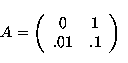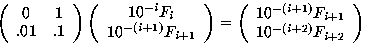## The Remarkable Number 1/89

by Robert Miner

The following remarkable fact was discovered by Cody Birsner, a student at the University of Oklahoma, in the fall of 1994. The discovery arose out of work for a term paper on the Fibonacci series.

### The Fibonacci Series

The Fibonacci series is a famous example of an object with a simple mathematical description, but a nearly inexhaustable list of bizarre and complicated properties. Originally, the series was described by Fibonacci in the 15th century in connection with the following problem:

Assume that a pair of rabbits will breed another pair of rabbits every month of their lives, after sexual maturity. Assume also that rabbits become sexually mature after two months. Starting with one newborn pair of rabbits, describe how many rabbits you get in successive months.

At the end of the first month you have 1 pair. After another month, you still have 1 pair. However, at the end of the third, the first pair has become sexually mature, and produced offspring, so you have 2 pair. At the end of the next month, the first pair reporduces again, while the second pair remains immature, so you have a total of 3 pair.

Proceeding in this fashion, one sees that the following list of numbers describes the breeding of the rabbits:

1,1,2,3,5,8,13,21,34,55,89,144,...

### Interesting Facts about the Fibonacci Series

• Each term in the sequence is the sum of the previous two. That is, 2 = 1 + 1, 3 = 2 + 1, 5 = 2 + 3, and so on.

• The Fibonacci series appears in a number of places throughout nature, for example, in the pattern in which sunflower seeds grow, and the leaf patterns of many plants.

• The successive ratios of the terms, i.e. 1/1, 2/1, 3/2, 5/3 ... tend to a number called the Golden Ratio by the Greeks. This number also appears thoughout nature, describing the spiral behavior of shells among other things. In addition, this number appears in many mathematical contexts, being related to pentagons and logarithmic spirals, to name a couple.

• The Fibbonaci series itself has many bizarre and curious properties, such as the one concerning 1/89 we are about to describe. In fact, there are so many of these strange interrelations, there is a mathematical journal called the Fibonacci Quarterly, entirely devoted to the series and its properties.

### The Punch Line

The decimal expansion of 1/89 is just the Fibonacci series, added together in an appropriate fashion.

Specifically, think of the Fibonacci series as being a sequence of decimal fractions, arranged so the right most digit of the nth Fibonacci number is in the n+1th decimal place. Then add:

```  .01
.001
.0002
.00003
.000005
.0000008
.00000013
.000000021
.0000000034
.00000000055
.000000000089
.0000000000144
.
.
+       .
----------------
.01123595505...
```
As you can easily check, 1/89 = .01123595595... Bizarre, eh?

## Sketch of Proof

This proof arose from discussions with Prof. Andy Magid and others in the math lounge at OU.Proof. Consider the matrixNote thatConsequently, it follows thatOn the other hand, by diagonalizing A, one easily checks that the geometric series converges, and in fact,ThusThe result now follows.